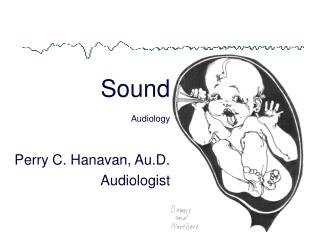DownloadDownload PresentationSound Audiology

Sound Audiology

Télécharger la présentationSound Audiology

- - - - - - - - - - - - - - - - - - - - - - - - - - - E N D - - - - - - - - - - - - - - - - - - - - - - - - - - -
Presentation Transcript

1. SoundAudiology Perry C. Hanavan, Au.D. Audiologist

2. Sound • Vibration • Perception

3. Propagation of Sound • Condensations • ____________ • Example of molecular motion • Components of sinusoid • Transverse wave simulation • Applet: Square, triangle, simulation

4. Components of Sound Physical Psychological ------------------------------------- • Intensity Loudness • Frequency ________ • Duration Length

5. Intensity • Decibel • Unit of measure of intensity • dB = 10logR (R=ratio)

6. Problem • Human hearing intensity dynamic range is quite broad • 1 Softest sound • 1,000,000,000,000 Loudest sound

7. Intensity Dynamic Range • 1 • 10 • 100 • 1000 • 10000 • 100000 • 1000000 • 10000000 • 100000000 • 1000000000 • 10000000000 • 100000000000 • 1000000000000 • 10000000000000 • 100000000000000

8. Honey I Shrunk the Kids • 1,3,5 or 1, 4, 8, 12 etc. reduces dynamic range somewhat • 1, 10, 100, 1000 shrinks a bit more • Yet, dynamic range is quite large

9. Solution • Ratio • Compare intensity value (number) to reference • 8:4 (really 2:1)

10. Aha! Ratio Compare 2 Numbers • 1/1 =1 • 10/1 =10 • 100/1 =100 • 1000/1 =1000 • 10000/1 • 100000/1 • 1000000/1 • 10000000/1 • 100000000/1 • 1000000000/1 • 10000000000/1 • 100000000000/1 • 1000000000000/1 • 10000000000000/1 • 100000000000000/1

11. Logarithms!! • Now that a ratio has been converted • Next step: Convert ratios to logs (base 10)

12. Lets Work with Logs!!! • 1 = 0 • 10 = 1 • 100 = 2 • 100000000000000 = ? • 14 • .1 = -1 • .01 = -2 • .00000000001 = ? • -11

13. So is that a decibel? • So far we have • 1. Converted a ratio to a number (10:1=10) • 2. Converted the ratio to a log (10=1) • We have created a ____ (A.G. Bell) • There are 10 decibels in a bel 2 bels = 20 decibels 8 bels = 80 decibels

14. WOW!!! • Decibel = 10 log _____ • A decibel consists of: • 1. Ratio • 2. Log • 3. X10

15. That was easy, let’s practice: • Integer: 10 X 10 = 100 • Log: 1 + 1 = 2 • Integer: 1000 X 1000 = ? • Log: 3 + 3 = 6 • Now take the log times 10 to convert to decibels

16. Not bad, let’s try this: • Integer: 100 / 10 = 10 • Log: 2 - 1 = 1 • Integer: 1000000 / 100 = ? • Log: 6 – 2 = 4 • Now take the log times 10 to convert to decibels

17. Ok, I can do that, but… • Some numbers are quite easy to work with in logs • However, some numbers you need to use a calculator or look in a CRC book of tables • Or you can memorize several numbers and calculate lots of logs • Hint: log = bel

18. Oh, one more thing: • Measures of ________ are often made in Sound Pressure Level (SPL) rather than Intensity Level (IL) • IL: amount of energy flowing thru a 1cm2 surface area • SPL: amount of pressure exerted on a 1cm2 surface area • IL= SPL2 • dBIL=10logR equals dBSPL=20logR

19. dB Intensity Level • Measure of energy • Reference 1.0 X 10 -16 watts/cm2 or .0000000000000001 watts/cm2

20. dB Sound Pressure Level • Measure of force or pressure • Reference 20 microPA

21. Sound Pressure Level 20/20 = 1:1 40/20 = 2:1 60/20 = 3:1 200/20 = 10:1 Intensity Level 1.0x10-16/1.0x10-16 2.0x10-16/1.0x10-16 3.0x10-16/1.0x10-16 4.0x10-16/1.0x10-16 1.0x10-15/1.0x10-16 Ratios in IL and SPL

22. dBIL=10logR or dBSPL=20logR Ratio No. Log dBIL dBSPL • 1:1 1 0 0 0 • 2:1 2 .3010 3.0 6.0 • 3:1 3 .4771 4.8 9.6 • 4:1 4 .6020 6.0 12.0 • 5:1 5 .6990 7.0 14.0 • 6:1 6 .7781 7.8 15.6 • 7:1 7 .8451 8.5 17.0 • 8:1 8 .9030 9.0 18.0 • 9:1 9 .9542 9.5 19.0 • 10:1 10 1.000 10.0 20.0

23. Can you do this? • Double SPL? • Double IL? • Triple SPL • Triple IL? • Quadruple SPL? • Quadruple IL?